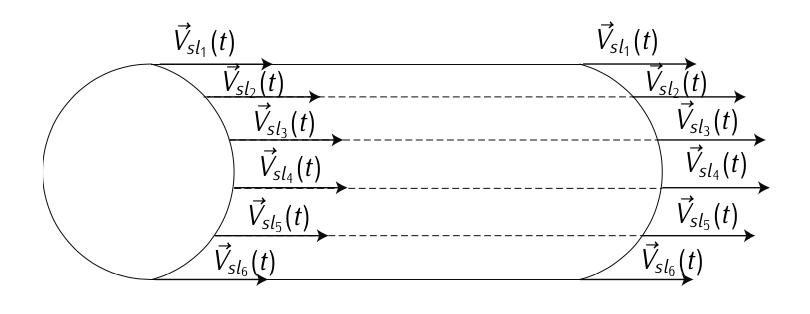# 3.1.1: Generalities

$$\newcommand{\vecs}{\overset { \rightharpoonup} {\mathbf{#1}} }$$ $$\newcommand{\vecd}{\overset{-\!-\!\rightharpoonup}{\vphantom{a}\smash {#1}}}$$$$\newcommand{\id}{\mathrm{id}}$$ $$\newcommand{\Span}{\mathrm{span}}$$ $$\newcommand{\kernel}{\mathrm{null}\,}$$ $$\newcommand{\range}{\mathrm{range}\,}$$ $$\newcommand{\RealPart}{\mathrm{Re}}$$ $$\newcommand{\ImaginaryPart}{\mathrm{Im}}$$ $$\newcommand{\Argument}{\mathrm{Arg}}$$ $$\newcommand{\norm}{\| #1 \|}$$ $$\newcommand{\inner}{\langle #1, #2 \rangle}$$ $$\newcommand{\Span}{\mathrm{span}}$$ $$\newcommand{\id}{\mathrm{id}}$$ $$\newcommand{\Span}{\mathrm{span}}$$ $$\newcommand{\kernel}{\mathrm{null}\,}$$ $$\newcommand{\range}{\mathrm{range}\,}$$ $$\newcommand{\RealPart}{\mathrm{Re}}$$ $$\newcommand{\ImaginaryPart}{\mathrm{Im}}$$ $$\newcommand{\Argument}{\mathrm{Arg}}$$ $$\newcommand{\norm}{\| #1 \|}$$ $$\newcommand{\inner}{\langle #1, #2 \rangle}$$ $$\newcommand{\Span}{\mathrm{span}}$$

Many of the fundamental laws of fluid mechanics apply to both liquid and gases. Liquids are nearly incompressible. Unlike a gas, the volume of a given mass of the liquid remains almost constant when a pressure is applied to the fluid. The interest herein is centered in gases since the atmosphere in which operate is a gas commonly know as air. Air is a viscous, compressible fluid composed mostly by nitrogen (78%) and oxygen (21%). Under some conditions (for instance, at low flight velocities), it can be considered incompressible.

Gas: A gas consists of a large number of molecules in random motion, each molecule having a particular velocity, position, and energy, varying because of collisions between molecules. The force per unit created on a surface by the time rate of change of momentum of the rebounding molecules is called the pressure. As long as the molecules are sufficiently apart so that the intermolecular magnetic forces are negligible, the gas acts as a continuos material in which the properties are determined by a statistical average of the particle effects. Such a gas is called a perfect gas.Figure 3.1: Stream line.

Stream line: The air as a continuos fluid flows under determined patterns1 confined into a finite space (the atmosphere). The curves tangent to the velocity vector of the flow at each point of the fluid in an instant of time are referred to as streamlines. Two streamlines can not cross each other except in points with null velocity, otherwise will mean that one point has two different velocities.Figure 3.2: Stream tube.

Stream tube: A stream tube is the locus2 of the streamlines which pass through a closed curve in a given instant. The stream tube can be though as a pipe inside the fluid, through its walls there is no flow.

1. the movement of a fluid is governed by the Navier-Stokes partial differential equations. The scope of this course does not cover the study of Navier-Stokes.

2. In geometry, a locus is a collection of points that share a property.

3.1.1: Generalities is shared under a CC BY-SA 3.0 license and was authored, remixed, and/or curated by Manuel Soler Arnedo via source content that was edited to conform to the style and standards of the LibreTexts platform; a detailed edit history is available upon request.